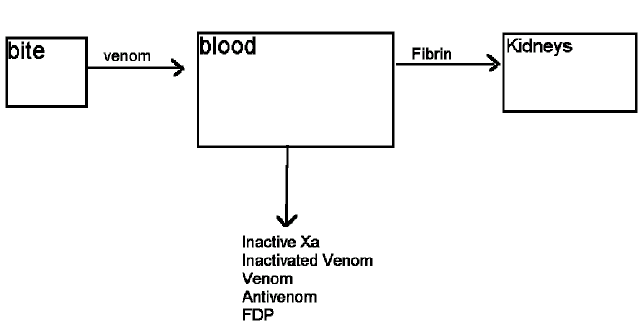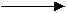Activation of Factor X:

Rvv +XXa

Rate constant = kx

-rx = kx(X)(RVV)

Formation of Fbrin:

Xa + FibrinogenFibrin

Rate Constant = kfib

Inactivation of Complex Xa:

Xa + ATIIIXa-ATIII

Rate Constant = kixa

Formation of Block:

Fibrin + Free siteBlock

Rate Constant = kdep

Digestion of Fibrin:

Fibrin + FDPSoluble products

Rate Constant = kfdp

Inactivation of Venom with antivenom:

Antivenom + VenomInactive venom

Rate Constant = krec